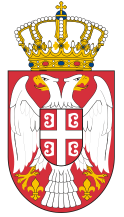Dokument se učitava

# Elements of Power SystemCourse code: 120307 | 6 ECTS credits

Basic information
Level of Studies: Undergraduate applied studies
Year of Study: 2
Semester: 3
Requirements: Knowledge of basic concepts in electrical engineering and mathematics.
Goal: To introduce students to the relevant theoretical and practical aspects of modelling and calculation of elements of power systems.
Outcome: Students will be able to calculate parameters necessary for designing elements of power systems.
Contents of the course
Theoretical instruction:
1. Basic characteristics of modern power systems and current state. Introductory considerations on the transmission of electricity.
2. Mechanical calculation of overhead lines. Equation of line of conductors. Climatic conditions for calculation.
3. The equation of change in the state of conductors. General and special cases.
4. Critical range and critical temperature. Examples.
5. Mechanical degree of safety of the transmission line. Mounting curves. Examples.
6. Pillars. Isolators. Pantry equipment. Arrangement of phase and protective conductors in the head of the pillar. Protective zone of protective conductors. Transposition.
7. Electric constants of a transmission line.
8. The phenomenon of the corona. A critical voltage of the corona. Loss due to the corona.
9. Electrical calculation of overhead lines. Equations of voltage spreading and transmission line currents with distributed parameters. Expansion constant and characteristic impedance.
10. Equivalent circuit of overhead lines. Calculation of symmetric simple-periodic and stable mode of operation of overhead lines.
11. Power transformer. Types and functional properties. Parameters and equivalent circuit. The principle of conversion the voltage, current and impedances to one voltage level.
12. Synchronous generator. Types and functions of the generator. Vector diagrams and replacement schemes of hydro and turbo-generators. Actual PQ diagram.
13. Basic characteristics and division of consumers.
14. Modern solutions of elements of electric power systems.
Practical instruction (Problem solving sessions/Lab work/Practical training):
1. Practical training program follows the lecture.
Textbooks and References
1. M. Đurić, Elementi elektroenergetskih sistema, Beopres, Beograd, 2009.
2. M. Đurić, Rešeni problemi iz elemenata elektroenergetskih sistema, Beopres, Beograd, 2000.
3. G. Dotlić, Elektroenergetika - kroz standarde, zakone, pravilnike i tehničke preporuke, SMEITS, Beograd, 2013.
Number of active classes (weekly)
Lectures: 3
Practical classes: 2
Other types of classes: 0
Grading (maximum number of points: 100)
Pre-exam obligations
Points
activities during lectures
10
activities on practial excersises
10
seminary work
20
colloquium
0
Final exam
Points
Written exam
60
Oral exam
0© 2019 School of Electrical and Computer Engineering of Applied Studies, Belgrade
Vojvode Stepe 283, office@viser.edu.rs, +381 11 2471 099